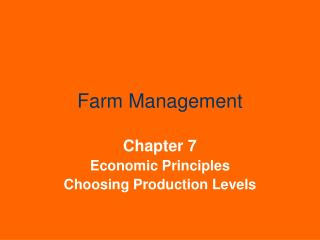DownloadDownload PresentationFarm Management

# Farm Management

Télécharger la présentation## Farm Management

- - - - - - - - - - - - - - - - - - - - - - - - - - - E N D - - - - - - - - - - - - - - - - - - - - - - - - - - -
##### Presentation Transcript

1. Farm Management Chapter 7 Economic Principles Choosing Production Levels

2. Chapter Outline • Marginalism • The Production Function • How Much Input to Use • How Much Output to Produce • Applying the Marginal Principles • Equal Marginal Principle

3. Chapter Objectives • To explain the concept of marginalism • To show the relation between a variable input and an output by use of a production function • To describe the calculation of average and marginal physical products • To illustrate the law of diminishing returns • To find the profit-maximizing point using the concepts of marginal value product and marginal input cost • To find the profit-maximizing point using the concepts of marginal revenue and marginal cost • To explain the use of the equal marginal principal

4. Marginalism The term marginal refers to incremental changes, either increases or decreases, that occur at the edge or at the “margin.” It may help to mentally substitute “extra” or “additional” whenever the word marginally is used. But keep in mind that the “extra” can be negative.

5. The Production Function The production function is a systematic way of showing the relation between different amounts of a resource or input that can be used to produce a product and the corresponding output.

6. Table 7-1 Production Function in Tabular Form

7. Total Physical Product Total physical product (TPP) is the amount of production expected from using each input level. Output or yield is often called total physical product.

8. Average Physical Product Average physical product (APP) is the average amount of output produced per unit of input used. APP = TPP input level

9. Marginal Physical Product Marginal physical product (MPP) is the additional TPP produced by using an additional unit of input. MPP = TPP input level

10. Figure 7-1 Graphical illustration of a production function

11. Stages of Production • Stage I: APP increasing, MPP>APP, TPP increasing • Stage II: APP decreasing, MPP<APP, TPP increasing • Stage III: TPP decreasing, MPP<0

12. Law of Diminishing Marginal Returns As additional units of a variable input are used in combination with one or more fixed inputs, marginal physical product will eventually begin to decline. Diminishing returns may start with the first unit of input used, or may start later after a period of increasing returns.

13. How Much Input to Use • Do not produce in Stage III, because more output can be produced with less input. • Do not normally produce in Stage I because the average productivity of the inputs continues to rise in this stage. • Stage II is the “rational stage” of production.

14. Marginal Value Product  total value product MVP =  input level TVP = TPP × product selling price If output price is constant: MVP = MPP × product selling price

15. Marginal Input Cost  total input cost MIC =  input level TIC = amount of input × input price If input price is constant: MIC = input selling price

16. Table 7-2 Marginal Value Product, Marginal Input Cost and the Optimum Input Level input price = \$12; output price = \$2

17. The Decision Rule MVP = MIC If MVP > MIC, additional profit can be made by using more input. If MIC > MVP, less input should be used.

18. How Much Output to Produce An alternative way to find the profit-maximizing point is to find directly the amount of output that maximizes profit.

19. Marginal Revenue  total revenue MR =  total physical product Total revenue = Total value product If output price is constant: MR = output selling price

20. Marginal Cost  total input cost MC =  total physical product

21. The Decision Rule MR=MC The decision rule, MR=MC, leads to the same point as the decision rule MVP=MIC.

22. Table 7-3 Marginal Revenue, Marginal Cost and the Optimum Output Level input price = \$12; output price = \$2

23. Applying the Marginal Principles Given prices for irrigation water and for corn, the principles from the last two sections can be used to determine the amount of water and the corresponding amount of corn that will maximize profit.

24. Table 7-4 Determining the Profit-Maximizing Irrigation Level for Corn Production water at \$3.00/acre-inch, corn at \$2.50/bu

25. Equal Marginal Principal In some situations an input may be limited so that the profit-maximizing point cannot be reached for all possible uses. A limited input should be allocated among competing uses in such a way that the marginal value products of the last unit used on each alternative are equal.

26. Table 7-5 Application of the Equal Marginal Principle to the Allocation of Irrigation Water

27. Figure 7-2 Illustration of the equal marginal system

28. Summary Economic principles using the concept of marginality provide useful guidelines for decision making. MVP and MIC are equated to find the profit-maximizing input level. MR and MC are equated to find the profit-maximizing output level. The equal marginal principle is used when a limited input must be allocated among competing uses.